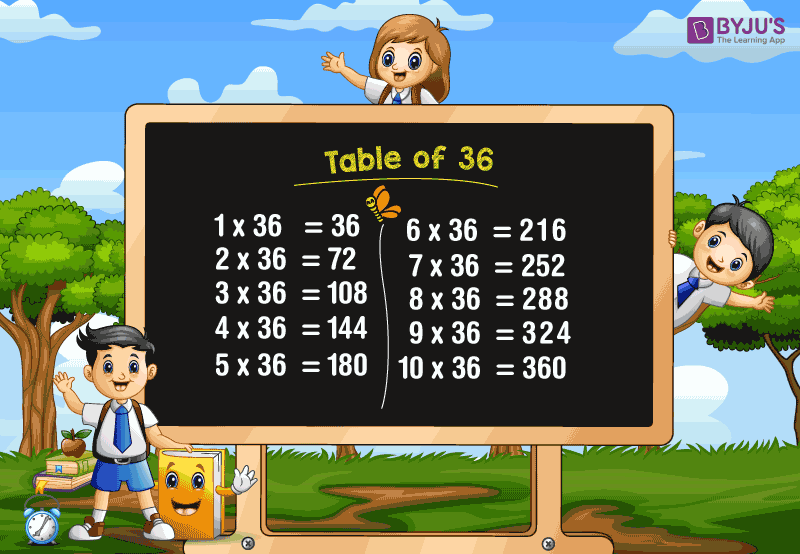# Table of 36

Table of 36 is the multiplication table that shows the multiplication of 36 with different whole numbers. Memorizing the table of 36 is important and is necessary for children’s education as it helps in building a strong foundation in the Mathematics subject. Here, we will learn the multiplication table of 36 with the help of a chart and learn how to read the 36 times table.

### Download Table of 36 Here## 36 Times Table

To get the table of 36, we need to multiply 36 with the different whole numbers. We know that multiplication is the repeated addition. In the 36 times table, we have to add the number “36” repeatedly.

For example, 36 × 2 = 72.

The same answer should be obtained using the repeated addition method. If we add 36 for two times, we get the same result.

(i.e.) 36+36 = 72.

Similarly, we can obtain the multiplication table of 36 easily.

Also, check tables from 1 to 100 for more details.

## Multiplication Table of 36

Go through the table given below to learn the multiplication of 36 with other numbers (from 1 to 20) to do fast mathematical calculations.

 36 × 1 = 36 36 × 2 = 72 36 × 3 = 108 36 × 4 = 144 36 × 5 = 180 36 × 6 = 216 36 × 7 = 252 36 × 8 = 288 36 × 9 = 324 36 × 10 = 360 36 × 11 = 396 36 × 12 = 432 36 × 13 = 468 36 × 14 = 504 36 × 15 = 540 36 × 16 = 576 36 × 17 = 612 36 × 18 = 648 36 × 19 = 684 36 × 20 = 720

### How to Read 36 Times Table?

Read the table of 36 as follows:

• One time thirty-six is 36
• Two times thirty-six is 72
• Three times thirty-six is 108
• Four times thirty-six is 144
• Five times thirty-six is 180
• Six times thirty-six is 216
• Seven times thirty-six is 252
• Eight times thirty-six is 288
• Nine times thirty-six is 324
• Ten times thirty-six is 360
• Eleven times thirty-six is 396
• Twelve times thirty-six is 432
• Thirteen times thirty-six is 468
• Fourteen times thirty-six is 504
• Fifteen times thirty-six is 540.

Get More Maths Tables:

## Frequently Asked Questions on Table of 36

### 1. What is the table of 36?

Table of 36 is the multiplication table that shows the multiplication of 36 with various whole numbers. In other words, if we add the number 36 repeatedly, we get the table of 36.

### 2. What is the value of 5 times 36?

5 times 36 is 180. (i.e.) 5 × 36 = 180.

### 3. What are the first 5 multiples of 36?

The first five multiples of 36 are 36, 72, 108, 144, 180.

### 4. What is the value of 36 times 10?

36 times 10 is 360. (i.e.) 36×10 = 360.

### 5. What is the value of 5 + (36×7)?

First, simplify the values inside the brackets.
We know that 36 times 7 is 252.
Hence, 5 + (36×7) = 5 + 252 = 257.